# GMAT Math : DSQ: Calculating the perimeter of a rectangle

## Example Questions

### Example Question #1 : Dsq: Calculating The Perimeter Of A Rectangle

What is the perimeter of rectangle?

(1) The area ofis 81

(2) Side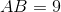Statement (1) ALONE is sufficient, but statement (2) alone is not sufficient.

EACH statement ALONE is sufficient.

Statement (2) ALONE is sufficient, but statement (1) alone is not sufficient.

BOTH statements TOGETHER are sufficient, but NEITHER statement ALONE is sufficient.

Statement (1) and (2) TOGETHER are NOT sufficient to answer the question asked, and additional data are needed.

BOTH statements TOGETHER are sufficient, but NEITHER statement ALONE is sufficient.

Explanation:

To know the perimeter of a rectangle, we need to know length and width.

Statement (1) provides Area, which does not provide the necessary information. NOT SUFFICIENT

Statement(2) provides one side, but we still need the other to determine perimeter. NOT SUFFICIENT.

Both together are sufficent however since if we know one side and the area, we can find the remaining side using division, and then use the two side lengths to find the perimeter.

### Example Question #2 : Dsq: Calculating The Perimeter Of A Rectangle

You are given a square and a rectangle. Which has the greater perimeter?

Statement 1: The length of the rectangle is twice the sidelength of the square,

Statement 2: The width of the rectangle is half the sidelength of the square.

BOTH statements TOGETHER are sufficient to answer the question, but NEITHER statement ALONE is sufficient to answer the question.

Statement 1 ALONE is sufficient to answer the question, but Statement 2 ALONE is NOT sufficient to answer the question.

EITHER statement ALONE is sufficient to answer the question.

BOTH statements TOGETHER are insufficient to answer the question.

Statement 2 ALONE is sufficient to answer the question, but Statement 1 ALONE is NOT sufficient to answer the question.

Statement 1 ALONE is sufficient to answer the question, but Statement 2 ALONE is NOT sufficient to answer the question.

Explanation:

Letbe the sidelength of the square. Then its perimeter is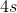.

From Statement 1 alone, the length of the rectangle is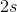, and, if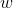is the width, the perimeter of the rectangle is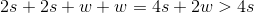. Therefore, we can prove that the rectangle has the greater perimeter.

From Statement 2 alone, the width of the rectangle is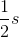, and its perimeter is at least- but unless we know the length, we do not know whether the total perimeter is greater than, equal to, or less than.

### Example Question #3 : Dsq: Calculating The Perimeter Of A Rectangle

The table in a hall has a length offeet. What is its perimeter?

I) The tabletop is exactly two and a half feet above the floor.

II) The area of the table is four times three more than the width.

Statement I is sufficient to answer the question, but statement II is not sufficient to answer the question.

Both statements are needed to answer the question.

Statement II is sufficient to answer the question, but statement I is not sufficient to answer the question.

Either statement is sufficient to answer the question.

Statement II is sufficient to answer the question, but statement I is not sufficient to answer the question.

Explanation:

To find perimeter we need length and width. We are given the length.

I) Is irrelevant.

II) We are given a way of relating area and width. Since we know that area is length times width, we can use II to set up an equation where we substitute in the known length along with the given statement to solve for our width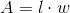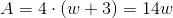Solve the second one for w and you're good to go!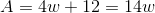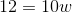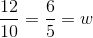.

Therefore the perimeter would be: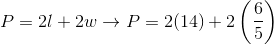### Example Question #4 : Dsq: Calculating The Perimeter Of A Rectangle

Farmer Jeff Jenkins is making a new rectangular field for his goats and needs to know how much fencing he needs to buy.

I) The goats will need at least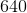square yards to roam.

II) One edge of the field will beyards long and will be made up of a river.

Both statements are needed to answer the question.

Statement I is sufficient to answer the question, but statement II is not sufficient to answer the question.

Either statement is sufficient to answer the question.

Statement II is sufficient to answer the question, but statement I is not sufficient to answer the question.

Both statements are needed to answer the question.

Explanation:

To find perimeter, we need the lengths of both sides.

I) Gives us the area, which gives us one equation and two unknowns. Alone this statement is not sufficient.

II) Gives us one side length of the field.

We can use this with I) to find the other side length.

Once we have both sides, we can find perimeter easily.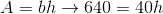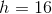Thus the perimeter is,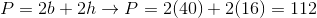.

### Example Question #5 : Dsq: Calculating The Perimeter Of A Rectangle

What is the perimeter of the rectangle?

1. The area is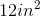.
2. The width measures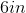.

Both statements taken together are sufficient to answer the question, but neither statement alone is sufficient.

Statement 2 alone is sufficient, but statement 1 alone is not sufficient to answer the question.

Each statement alone is sufficient to answer the question.

Statements 1 and 2 are not sufficient, and additional data is needed to answer the question.

Statement 1 alone is sufficient, but statement 2 alone is not sufficient to answer the question.

Both statements taken together are sufficient to answer the question, but neither statement alone is sufficient.

Explanation:

Statement 1: Although the area is given, we need more information in order to answer the question.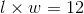but this can means our dimenions are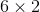or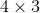Statement 2: We're told the width measureswhich means our dimensions are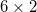Using BOTH statements, we can find the perimeter: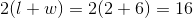### Example Question #6 : Dsq: Calculating The Perimeter Of A Rectangle

True or false: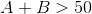Statement 1: A rectangle with lengthand widthhas area greater than 100.

Statement 2: A rectangle with lengthand widthhas perimeter greater than 100.

STATEMENT 2 ALONE provides sufficient information to answer the question, but STATEMENT 1 ALONE does NOT provide sufficient information to answer the question.

BOTH STATEMENTS TOGETHER do NOT provide sufficient information to answer the question.

BOTH STATEMENTS TOGETHER provide sufficient information to answer the question, but NEITHER STATEMENT ALONE provides sufficient information to answer the question.

STATEMENT 1 ALONE provides sufficient information to answer the question, but STATEMENT 2 ALONE does NOT provide sufficient information to answer the question.

EITHER STATEMENT ALONE provides sufficient information to answer the question.

STATEMENT 2 ALONE provides sufficient information to answer the question, but STATEMENT 1 ALONE does NOT provide sufficient information to answer the question.

Explanation:

Statement 1 alone provides insufficient information. For example:

Case 1: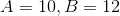The area of a rectangle with these dimensions is their product, which is 120; this exceeds 100.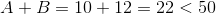Case 2: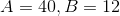The area of a rectangle with these dimensions is their product, which is 480; this exceeds 100.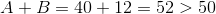Assume Statement 2 alone. The perimeter of a rectangle is twice the sum of their dimensions, so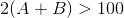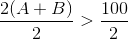, answering the question in the affirmative.

Tired of practice problems?

Try live online GMAT prep today.